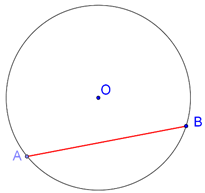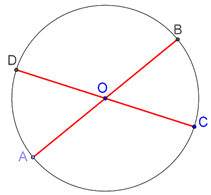# Chords and Diameters

Chords and Diameters

A chord of a circle is any line segment joining two points on the circumference of the circle. In the following figure, AB is a chord of the circle drawn:A chord which passes through the center of the circle is known as a diameter chord, or simply a diameter. In the following figure, AB and CD are two diameter chords of the circle drawn:Circles
grade 9 | Questions Set 2
Circles
grade 9 | Questions Set 1
Circles
Circles
More Important Topics
Numbers
Algebra
Geometry
Measurement
Money
Data
Trigonometry
Calculus
More Important Topics
Numbers
Algebra
Geometry
Measurement
Money
Data
Trigonometry
Calculus